Home
 Introduction to Decimals - symbols (Conceptual understanding increases success.) .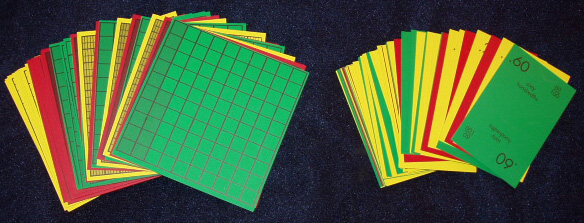Materials: Deck of Decimal Squares and Deck of Decimal Playing Cards for each group Decimal Symbolism . Point out that we have seen examples of games and activities that involved the concepts of equality and inequality and which did not require the use of decimal symbolism. Discuss the need for these types of activities to develop conceptual understanding before symbols and rules are introduced. Then place the following transparency on the overhead which calls for the use of models for teaching rational numbers.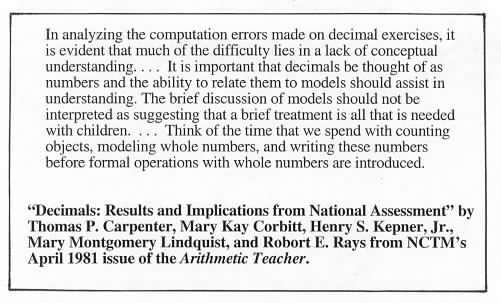Write the decimals .3 and .325 on the overhead and ask for descriptions of the Decimal Squares for these decimals. Ask for the names of these decimals: three-tenths or point three; etc.. Discuss the abstractness of decimal notation. Write the fractions 3/10 and 325/1000 on the overhead and note that these symbols show both the total number of equal parts in a whole and the number of shaded parts whereas the decimal notation shows a number for the shaded parts but no number for the total number of parts. This brevity and conciseness of decimal notation is one cause of student difficulty in creating visual images and meaning for decimals. Note that .3 and 3/10 have the same name "three-tenths" but that .3 is also called "point three". Similarly and 65/100 are both called "sixty-five hundredths" but .65 is also called "point sixty-five" etc..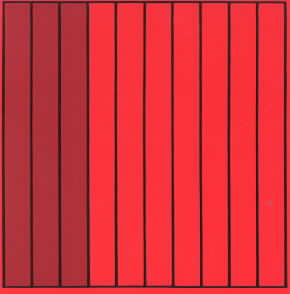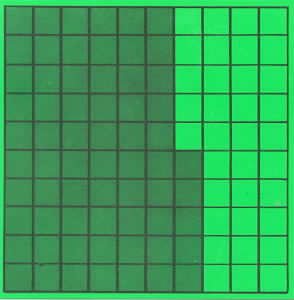.3 or 3/10 .65 or 65/100 .325 or 325/1000 Discuss the following: (a) Decimals for the red squares have only one place because the number of shaded parts is a single digit number. Ask people to count by tenths . . . .9 and put a red square for .9 on the overhead to show that the next number after .9 when counting by tenths is 1.0 for a whole square and zero tenths. Discuss the tendency for students to write .10 following .9 but that the squares can be used to show that .10 is equal to .1 by using squares with 10 shaded parts out of 100 and 1 shaded part out of 10.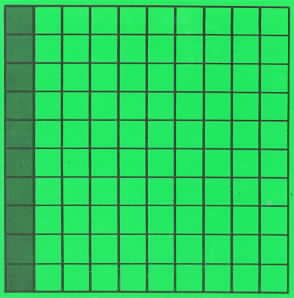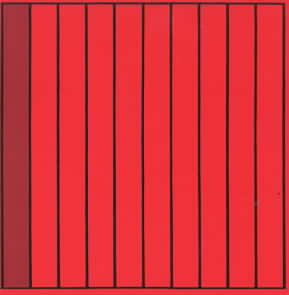. (b) Decimals for the green squares have two places because two-digit numbers are needed to describe the number of shaded parts. Ask people to count by hundredths beginning with .92 and counting on. Put a green square for .95 on the overhead and shade the additional parts for .96 and .99 to show that the next number after .99 when counting by hundredths is 1.00 for a whole square and zero hundredths.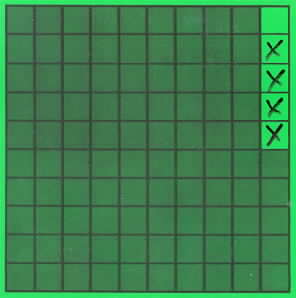(c) Decimals for the yellow squares have three places because three-digit numbers are needed to describe the number of shaded parts. Ask people to count by thousandths beginning with .996 and counting on. Put a yellow square for .950 on the overhead and shade the additional parts for .996 and .999 to show that the next number after .999 when counting by thousandths is 1.000 for a whole square and zero thousandths.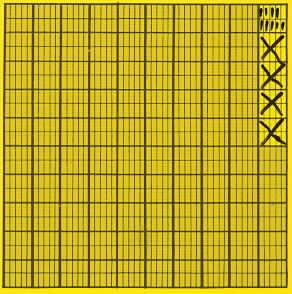Connecting Decimals to Models Ask each group to look at the Decimal Playing Cards and to list some observations.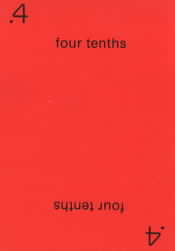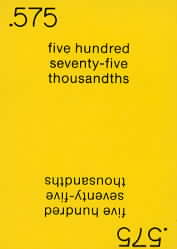Here are a few possibilities: the cards are colored coded to match the squares; and there is a decimal for each square. Point out that the color coding helps to provide a gradual transition to the use of decimals because the color suggests the total number of parts in a square and the student only needs to look at the whole number part of the decimal to determine the number of shaded parts for the square. Activities for Introducing Decimals "Here are two activities that can be used to introduce decimals: (1) Squares to Decimals – Each person selects a square finds its playing card and writes its fraction; (2) Decimals to Squares – Each person selects a playing card and then writes a description of its square. Game of MATCH This is a good game to gain familiarity with decimals (see rules below). This game is also on page 71 of the Decimal Squares Teacher’s Guide. Playing DECIMAL SQUARES BINGO using the colored squares on the mats and the decimal playing cards is another way to reinforce the connection between the model and decimal symbols .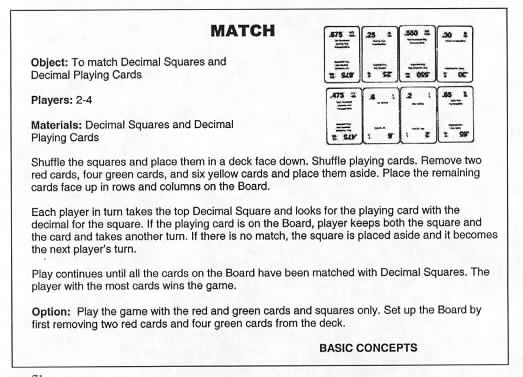In the following version of Match the students have placed the squares face up in rows and columns and are taking turns matching the decimals from their playing cards to the corresponding squares.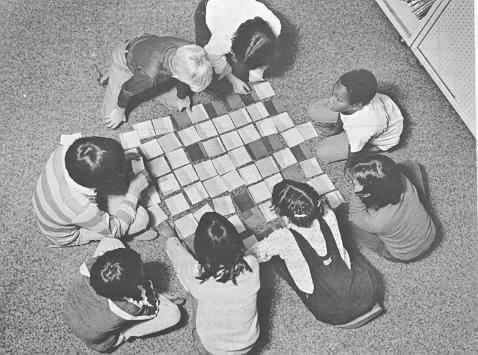Photo courtesy of Herb Moyer